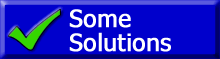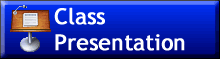ChaptersThis text is meant to accompany class discussions. It is not everything there is to know about the forces. It is meant as a  prep for class. More detailed notes and examples are given in the class notes, presentations, and demonstrations (click here.)

 Page Description 1 Introduction, Forces: Weight, Normal Force, Friction, Tension, Net Force, Online Review Quiz 2 Using Free Body Diagrams to develop a math model How to draw free body diagrams 3 Going from diagram to math model. 6 Examples and an online review quiz 3b More free body diagram examples 4 More examples

Objectives

Student will be able to:

• Define “body”
• Identify what forces are acting on a body
• Define the following forces using a description and formulas
• Weight
• Normal force
• Frictional force
• Kinetic Friction
• Static Friction
• Tension
• Net Force
• Identify when the forces above are acting on a body AND describe their direction of influence
• Draw a free body diagram (“fbd”) using the skills and definitions above.Class note and example problems with solutionsby Tony Wayne ...(If you are a teacher, please feel free to use these resources in your teaching.)